﻿ 双调和抛物方程的加权Lp估计 Weighted Lp Estimates for the Bi-Harmonic Parabolic Equation

Pure Mathematics
Vol.05 No.02(2015), Article ID:14904,7 pages
10.12677/PM.2015.52007

Weighted Lp Estimates for the Bi-Harmonic Parabolic Equation

Huacui Yu

College of Sciencers, Shanghai University, Shanghai

Email: 15202161183@163.com

Received: Feb. 18th, 2015; accepted: Feb. 27th, 2015; published: Mar. 3rd, 2015

Copyright © 2015 by author and Hans Publishers Inc.ABSTRACT

Schauder estimates and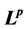estimates are the basic regularity estimates in the partial differential equations. In this paper we mainly study a new class of regularity estimates, weightedestimates for the bi-harmonic parabolic equation.

Keywords:Bi-Harmonic Parabolic Equation, Weighted,Estimates, Regularity Estimates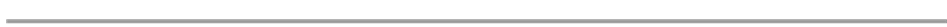Email: 15202161183@163.com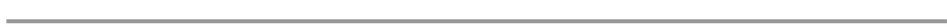Schauder估计和估计是偏微分方程基本的正则性估计。本文我们主要研究双调和抛物方程的一类新的正则性估计——加权估计。1. 引言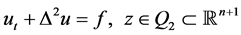(1.1)(1.2)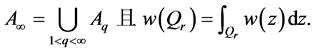2) 如果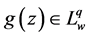，那么，任意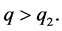3) 如果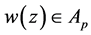，那么，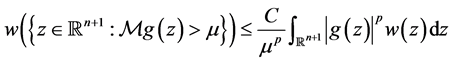4) 如果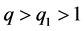，那么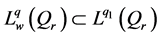(1.3)(1.4)

2. 主要结论的证明(2.1)(2.2)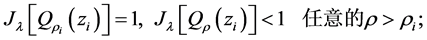(2.3)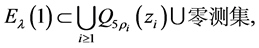(2.4)(2.5)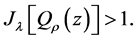(2.6)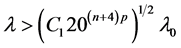时，进一步可得：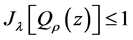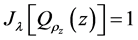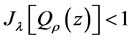任意的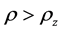2) 由(2.2)和(2.3)，可得：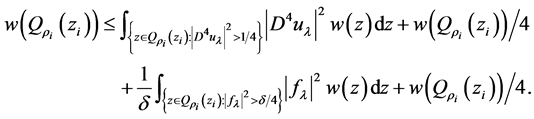(2.7)(2.8).估计(见 )，我们可得：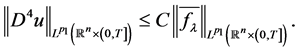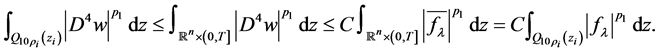(2.9)，可知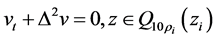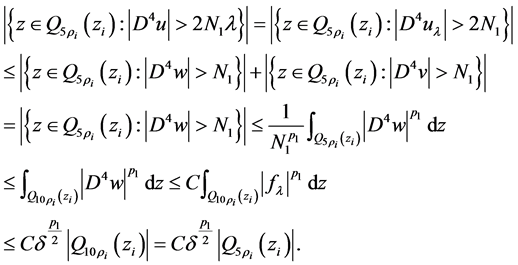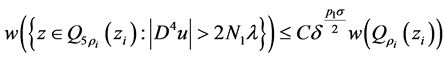(2.10)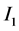的估计。事实上，首先可得下面不等式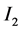的估计，由于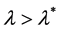，利用(2.10)及注1.2中的(2)，可得Weighted Lp Estimates for the Bi-Harmonic Parabolic Equation. 理论数学,02,46-53. doi: 10.12677/PM.2015.52007

1. 1. Wang, L. (2003) A geometric approach to the Calderón-Zygmund estimates. Acta Mathematica Sinica (Engl. Ser.), 19, 381-396.

2. 2. Caffarelli, L. and Peral, I. (1998) On W1,p estimates for elliptic equations in divergence form. Commu-nications on Pure and Applied Mathematics, 51, 1-21.

3. 3. Byun, S. and Wang, L. (2004) Elliptic equations with BMO coefficients in Reifenberg domains. Communications on Pure and Applied Mathematics, 57, 1283-1310.

4. 4. Byun, S. and Wang, L. (2005) Lp estimates for parabolic equations in Reifenberg domains. Journal of Functional Analysis, 223, 44-85.

5. 5. Byun, S. and Wang, L. (2005) Parabolic equations in Reifenberg domains. Archive for Rational Mechanics and Analysis, 176, 271-301.

6. 6. Byun, S. andWang, L. (2007) Parabolic equations in Time Dependent Reifenberg domains. Advances in Mathematics, 212, 797-818.

7. 7. Byun, S. andWang, L. (2007) Quasilinear elliptic equations with BMO coefficients in Lipschitz domains. Transactions of the American Mathematical Society, 359, 5899-5913.

8. 8. Mengesha, T. and Phuc, N. (2011) Weighted and regularity estimates for nonlinear equations on Reifenberg flat domains. Journal of Differential Equations, 250, 2485-2507.

9. 9. Mengesha, T. and Phuc, N. (2012) Global estimates for quasilinear elliptic equations on Reifenberg flat domains. Archive for Rational Mechanics and Analysis, 203, 189-216.

10. 10. Byun, S., Palagachev, D. and Ryu, S. (2013) weighted W1,p estimates for solutions of non-linear parabolic equations over non-smooth domains. Bulletin of the London Mathematical Society, 45, 765-778.

11. 11. Yao, F.P. (2014) Weighted gradient estimates for the general elliptic p(x)-Laplacian equations. Journal of Mathematical Analysis and Applications, 415, 644-660.

12. 12. Yao, F.P. (2014) Weighted Lp estimates for the elliptic Schrödinger operator. Journal of Mathematical Analysis and Applications, 33, 1-13.

13. 13. 周民强 (2003) 调和分析讲义(实变方法). 北京大学出版社, 北京.

14. 14. Acerbi, E. and Mingione, G. (2007) Gradient estimates for a class of parabolic systems. Duke Mathematical Journal, 136, 285-320.

15. 15. Byun, S., Yao, F.P. and Zhou, S.L. (2008) Gradient estimates in Orlicz space for nonlinear elliptic equations. Journal of Functional Analysis, 255, 1851-1873.

16. 16. Byun, S. and Ryu, S. (2013) Global weighted estimates for the gradient of solutions to nonlinear el-liptic equations. Annales de l’Institut Henri Poincare (C) Non Linear Analysis, 30, 291-313.

17. 17. Wang, L.H., Yao, F.P., Zhou, S.L. and Jia, H.L. (2009) Optimal regularity for the poisson equation. Proceedings of the American Ma-thematical Society, 137, 2037-2047.

18. 18. Yao, F.P. and Zhou, S.L. (2008) Global Lp estimates for the parabolic equa-tion of the Bi-harmonic type. Journal of Partial Differential Equations, 21, 315-334.

19. 19. Evans, L. (1997) Partial differential equations. American Mathematical Society, Providence.

20. 20. 陈亚浙, 吴兰成 (1997) 二阶椭圆型方程与椭圆型方程组. 科学出版社, 北京.